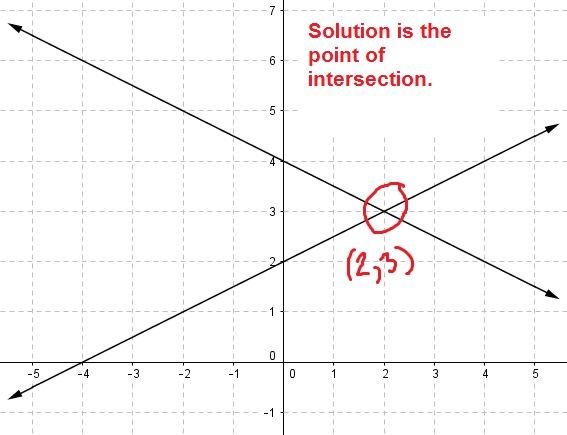# Solving systems of equations by graphing

While systems of two linear equations with two unknowns can be solved using algebra, it is also possible to systems of equations by graphing each equation in the system. In the examples below, you will see how to find the solution to a system of equations from a graph, how to determine if there are no solutions, and how to determine if there are infinitely many solutions. You will also see an example of nonlinear systems and its graph.

## The graph of a system of equations with with one solution

Consider the following system of equations.

$$\begin{array}{l} x+2y=8\\ \\x-2y=-4\end{array}$$

If you solve this system using the addition method, you will find that $$x = 2$$ and $$y = 3$$. But, what does this look like on a graph?Notice that the two lines intersect at exactly one point, and that this point is $$(2, 3)$$. For any system with one solution, the graphs will intersect at one point and this point will represent the solution to the system of equations.

If you don’t remember how to graph these equations, make sure to check this article: how to graph linear equations.

## The graph of a system of equations with no solutions

The following system of equations has no solution.

$$\begin{array}{l} x-y=-2\\ \\x-y=1\end{array}$$

How would you know? One way would be to try and solve the system, and see that you get an untrue statement. Another would be to realize that the first equation is saying that x–y is one number, while the second equation is saying that x–y has a different value. These can’t both be true. The difference must have a single value.

You could also tell by looking at the graph of the system of equations.The two lines are parallel, meaning that they will never intersect. For any system of equations, if there is no solution the the system, the two graphs will not intersect at any point. For linear equations, this will result in a graph of two parallel lines.

## Infinitely many solutions.

Let’s look now at a system of equations with infinitely many solutions. While it will not always be so obvious, you can tell that this system has infinitely many solutions because the second equation is just a multiple of the first.

$$\begin{array}{l} x+y=-2\\ \\2x+2y=-4\end{array}$$

If you were to graph these two equations, you would get the following result.Even though the system of equations includes two linear equations, you end up with a single line. This will always be the case when there are infinitely many solutions. The solution set is actually all points along the line.

## Can there be two or three or four solutions?

You may have noticed that we covered only three cases: one solution, no solutions, and infinitely many solutions. When working with linear equations, these are the only possibilities.

This is because the graphs of the equations are lines. A straight line can only intersect another straight line at one point, at no points, or at all points (they are just the same line). If two lines intersected at, say exactly two points, then the lines would have to bend and would no longer be lines.

If you are working with nonlinear equations, however, things are quite different! For example, in the graph below, the circle and the line intersect at exactly two points.These two points would represent solutions to the system of equations. But again, this is only because both graphs are not from a linear equation.